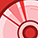Level 1Level 1

# DEMO POSITION2GO - COM port does not work when two arrays do calculations or assignation

Hello
I'm using the DEMO POSITION2GO radar, and I'm trying to develop my own algorithm with DAVE IDE.
The COM port will not work when two arrays do calculations or assignation.
But the build is successful.
Below is the code I found problematic.

1.do assignation

double _Complex x1 = {};

getdata()

{

double _Complex rx12 = {{0.5008547 + 0.5177045*I,0.6893773 + 0.5008547*I, ...... };
int i, j;
for(i=0;i<2;i++){
for(j=0;j<128;j++){
x1[i][j] = (rx12[i][j]-0.5-0.5*I)* 2；//Doing this line will cause the COM port to not be read
}
}
}

2.do calculations

void fft1
(double _Complex x[],int pekora)     /*fft1(x1,0); double _Complex x1={{0.5008547 + 0.5177045*I,0.6893773 + 0.5008547*I,0.9377289......};*/
{
double _Complex fev={};
double _Complex F64 = {{1.000000 + 0.000000*I,1.000000 + 0.000000*I,......};
double _Complex f;
int i, j,g;

P = 128/2；
if(pekora == 0){
if(P==64){
for(g=0;g<16;g++){
for(i=0;i<213;i++){
for(j=0;j< P;j++){
f = F64[i][j]*x[g][2*j];     //Doing this line will cause the COM port to not be read
fev[i] = fev[i]+f;
}
}
}
}
}
}

I don't know why the USB COM port is not being read.

Can you give me some advice to fix it?

Any help would be very welcome.

thanks

2 RepliesModeratorModerator

# Re: DEMO POSITION2GO - COM port does not work when two arrays do calculations or assignation

Hi @_Tom_ ,

Does the COM port hang up after one read or doesn't read at all? Where are you storing the raw_data?
Could you share the project, so that I can get more insight into the problem.

Thanks and regards,
YashrajLevel 1Level 1

# Re: DEMO POSITION2GO - COM port does not work when two arrays do calculations or assignation

Hi @PalY

The COM port doesn't read at all.

Because my algorithm is based on matlab,so I need the raw data in complex style.

But I don't know how to get complex raw data like matlab in DAVE IDE.

Then I made a double _Complex array to store the complex raw data taken from extract_raw_data.m,and check other functions for errors in DAVE IDE

I share the project algo.zip,Could you help me find out what's wrong.

Thank you.